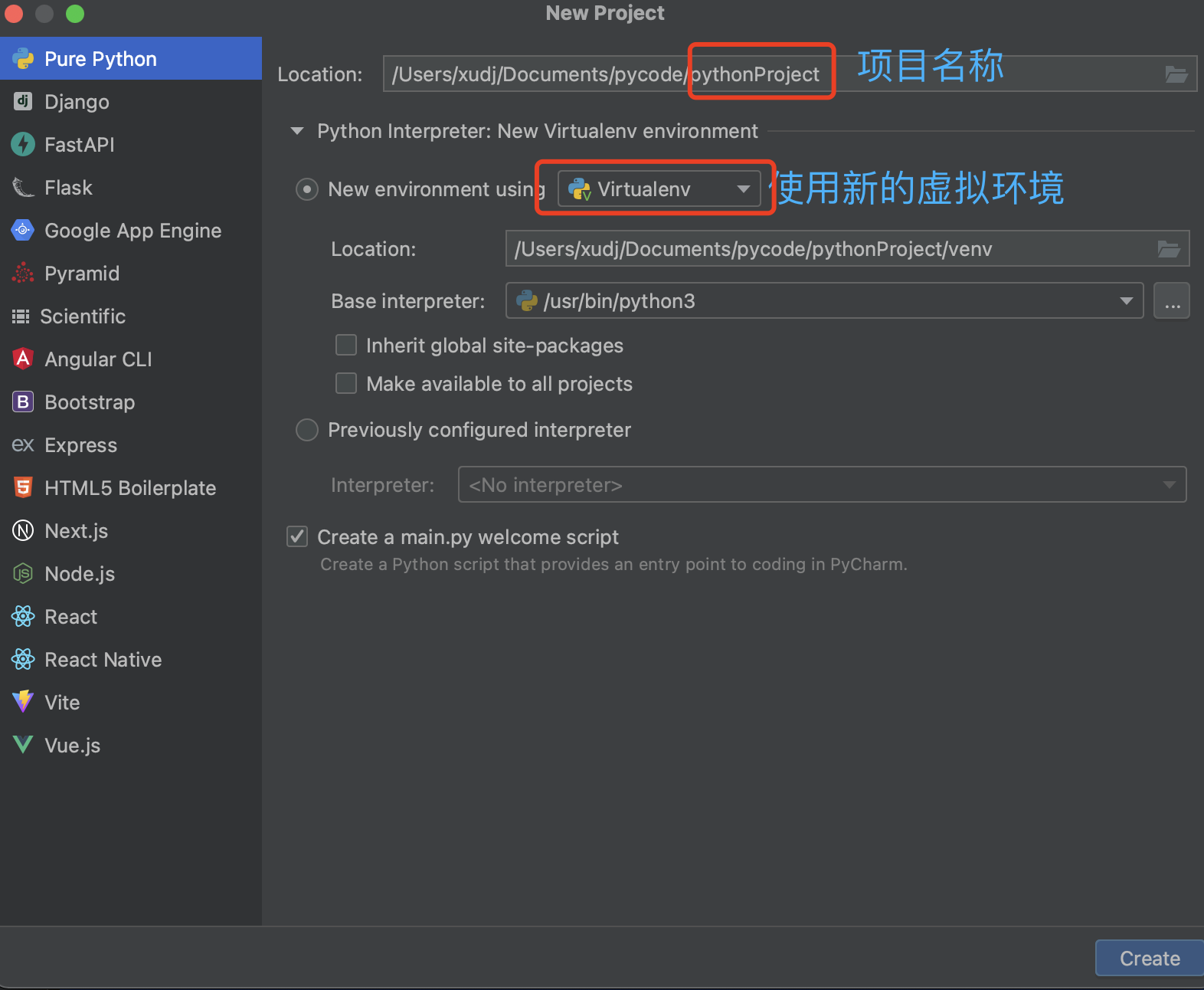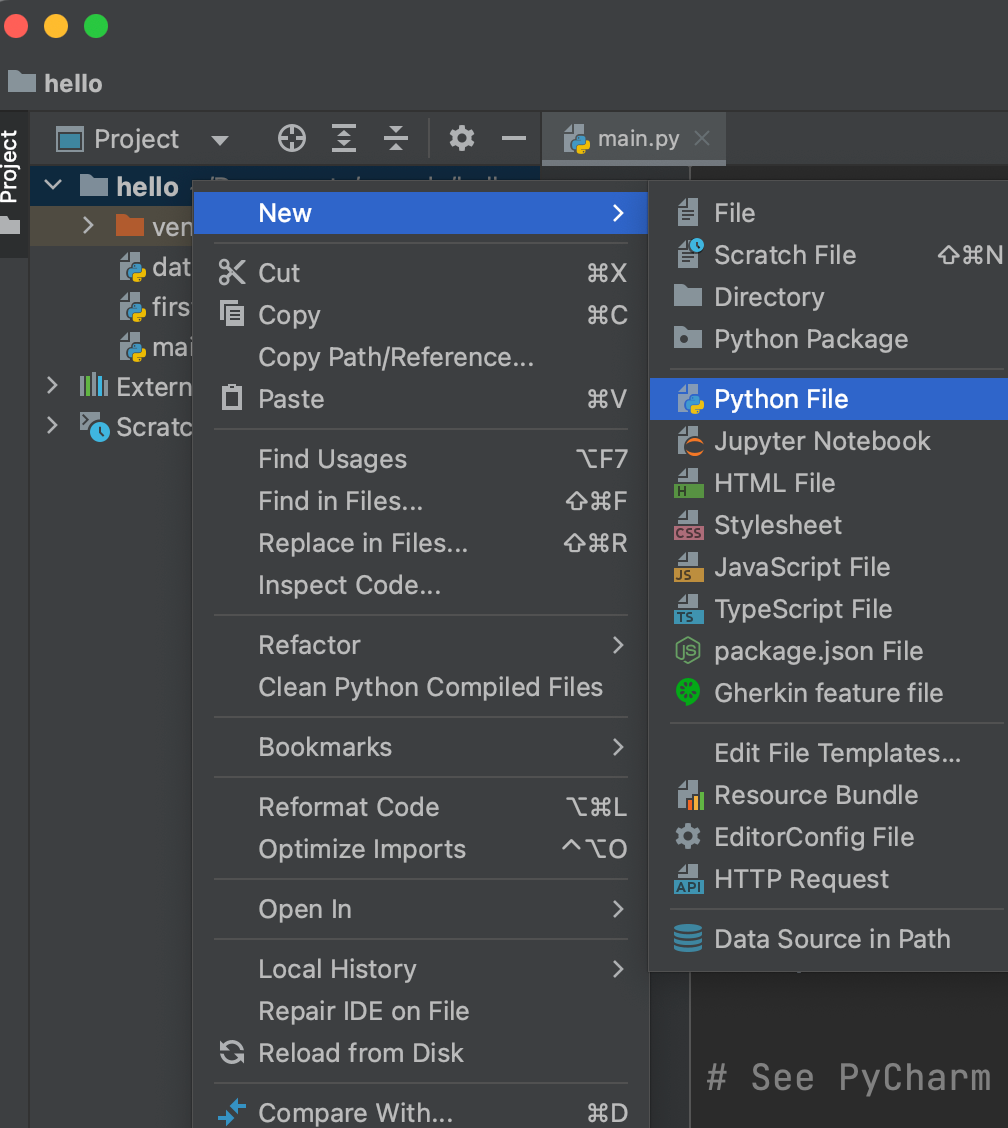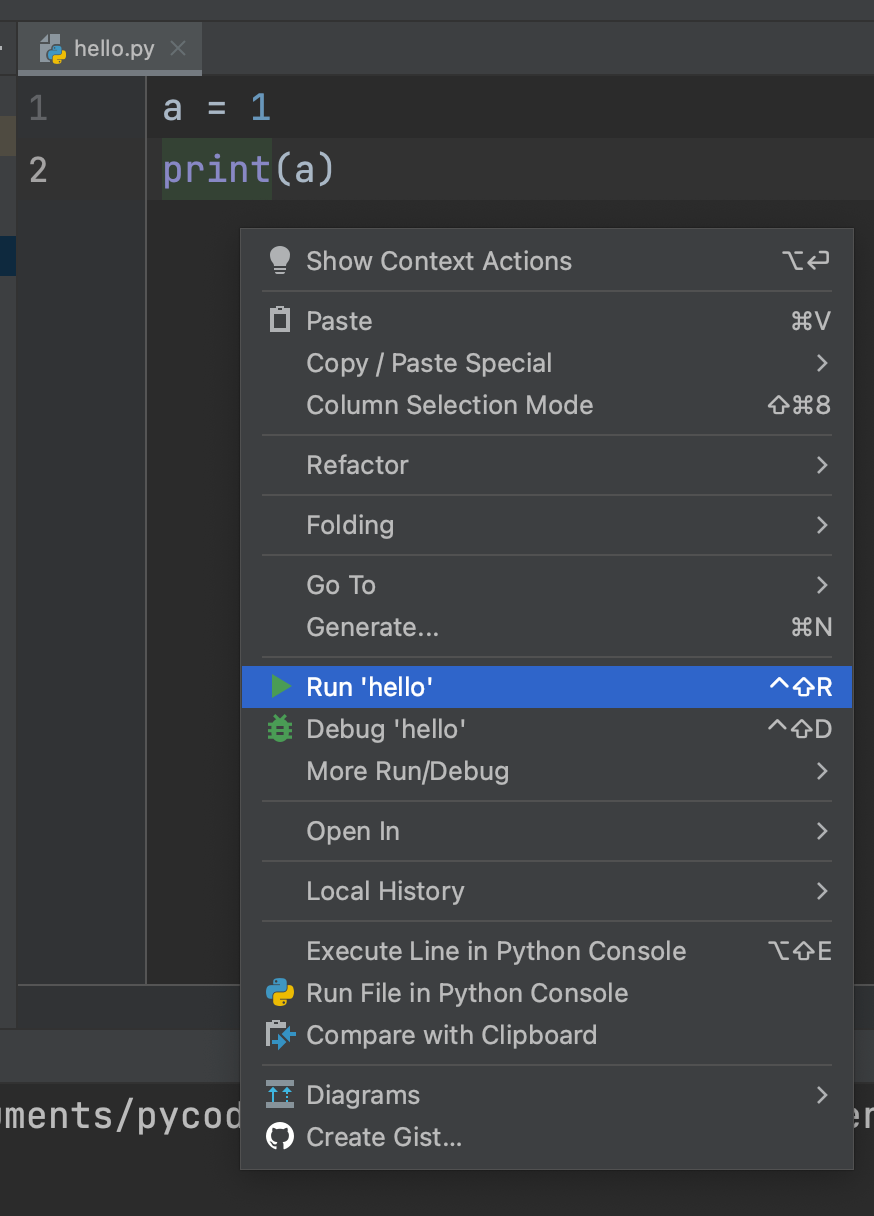• 累计撰写 83 篇文章
• 累计创建 150 个标签
• 累计收到 5 条评论

### 目 录CONTENT# python入门学习2023-01-31 / 0 评论 / 3 点赞 / 279 阅读 / 1,417 字

## 前言

python是在1990 年初开发的一种解释型编程语言。

（除编译型和解释型语言外，还有一种半编译半解释型语言，比如 Java 和 C# 等，这类语言将源代码先转换成一种中间文件（字节码文件），然后再将中间文件拿到虚拟机中执行。）

Python 语言最大的特点就是简单，实现代码比较简短。

## 本地环境安装

1、首选安装本地的python环境

> python3 --version
Python 3.10.9


2、修改环境变量

# python3
alias python=/opt/homebrew/bin/python3
alias pip=/opt/homebrew/bin/pip3
export PATH=\$PATH:/opt/homebrew/bin/


> python --version
Python 3.10.9


## hello world

1、使用命令行：python，进入python环境：

>>> a = 1
>>> print(a)
1


2、使用python编辑器：PyCharm## 基础语法与数据结构

### 基础语法

1、python是弱类型语言，定义变量时无需标明类型

str='123456789'
print(str)


2、使用缩进表示代码块，不用大括号

if True:
print ("True")
else:
print ("False")    # 缩进不一致，会导致运行错误


3、一行写不下，用反斜杠 \ 实现多行语句

4、print内置输出函数，默认是换行的，如果要实现不换行需要在变量末尾加上 end=“”

x = "a"
y = "b"
# 不换行输出
print( x, end=" " )
print( y, end=" " )


### 数据结构

1、字符串

var1 = 'hello'
var2 = 'world'
print(var1 + ' ' + var2)  # 输出：hello world

# 字符串截取，[x:y]，左闭右开原则
var1 = var1[0:2]
print(var1)  # 输出：he



2、数字

number = 0xFF  # 十六进制
print(number)  # 255
number = 0o37  # 八进制
print(number)  # 31

# 数字类型转换
x = 1.0
print(x)  # 1.0
print(int(x))  # 1



3、列表

list1 = ['a', 'b', 'c', 'd']
list2 = [1, 2, 3, 4]
print(list1)  # a
print(list1)  # c

# 列表截取，和字符串一样，使用[:]
list3 = list1[1:2]
print(list3)  # ['b']

# 取列表最大值、最小值、长度等
print(len(list1))  # 4
print(min(list2))  # 1



4、元组

tup1 = ('a', 'b', 'c', 'd')
tup2 = 1, 2, 3, 4  # 可以省略小括号
print(tup1)  # ('a', 'b', 'c', 'd')
print(type(tup2))  # <class 'tuple'>
print(tup2)  # (1, 2, 3, 4)

# 只有一个元素时，需要后面加个逗号，否则会把括号当成运算符, 如tup1 = (10)
tup1 = (10,)
print(type(tup1))  # <class 'tuple'>

# 和元素一样，可以按[:]截取，也可以按索引访问
tup3 = tup2[2:3]
print(tup3)  # (3,)



5、字典
key:value键值对；key唯一不可变；value可以任意类型

dict1 = {'key1': 'value1', 2: 2, 'key3': 'value3'}
print(dict1)  # {'key1': 'value1', 2: 2, 'key3': 'value3'}
print(len(dict1))  # 3

# 访问字典里的值
print(dict1)  # 2
print(dict1['key1'])  # value1

# 修改和删除
dict1 = 3
del dict1['key3']
print(dict1)  # {'key1': 'value1', 2: 3}

# 键必须不可变，所以可以用数字，字符串或元组充当，而用列表就不行
# tinyDict = {['Name']: 'Run', 'Age': 7}  # TypeError: unhashable type: 'list'



6、集合

set1 = {'a', 'b', 'c'}
set2 = set((1, 2, 3))
print(set1)  # {'c', 'a', 'b'}
print(set2)  # {1, 2, 3}

# 判断元素是否在集合中
flag = 'a' in set1
print(flag)  # True


## 总结

python入门，语言是相同的，一些语法、数据结构和使用会不太一样，后续多实践，边用边学

3